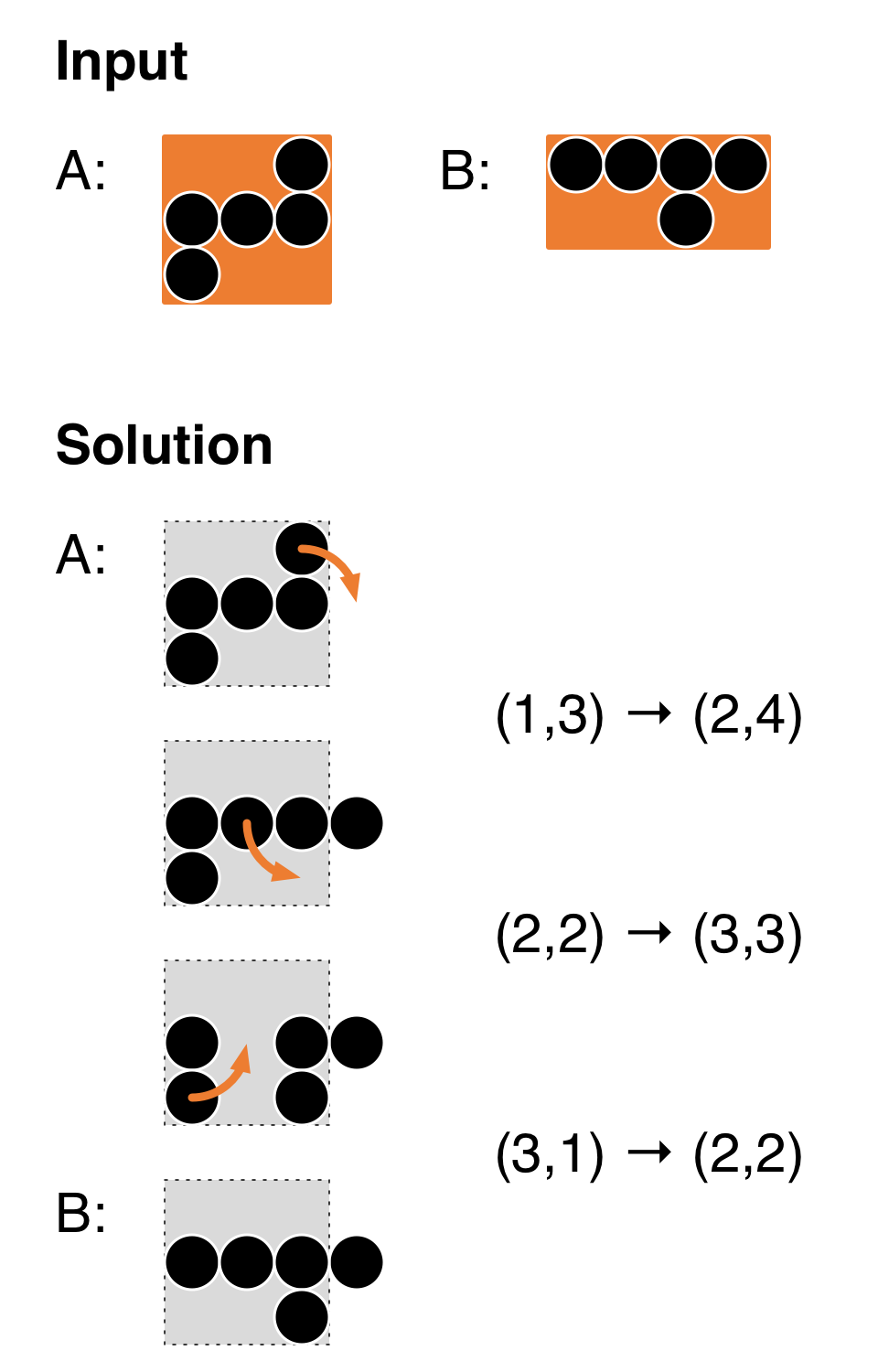CSES - HIIT Open 2017 - Impatience
• Time limit: 2.00 s
• Memory limit: 512 MB

Impatience is a one-person game played on an infinitely large 2-dimensional grid. Each grid cell is either empty or it contains one pebble. We refer to grid cells with coordinates (r,c), where r is the row (numbered from top to bottom) and c is the column (numbered from left to right).

You are given two shapes, A and B, that show the positions of the pebbles. In the beginning, you place all pebbles according to shape A, with the top left corner in cell (1,1). Then you can perform a sequence of valid rotations in which you move one pebble to a new position. You win if you find a sequence of rotations so that your final configuration looks like shape B (possibly shifted horizontally and vertically, but not rotated or mirrored).

A valid rotation is defined as follows. Let r_1 and r_2 be two adjacent rows (i.e., |r_1-r_2| = 1) and let c_1 and c_2 be two adjacent columns (i.e., |c_1-c_2| = 1). Now assume that:

• (r_1,c_1) contains a pebble
• (r_2,c_2) is empty
• exactly one of (r_1,c_2) and (r_2,c_1) contains a pebble.

Then we can move a pebble from (r_1,c_1) to (r_2,c_2); such a move is denoted by (r_1,c_1) \to (r_2,c_2). See the figure below for an example of inputs and a sequence of 3 valid rotations that wins the game.Note that you are free to use both negative and positive coordinates. You can freely step outside the boundaries of the images A and B.

# Input

As input, you will receive two shapes. Each shape is encoded as follows:

• One line with two numbers n and m: the height and the width of the shape.
• n lines, with m characters on each line. The characters are either 0 or 1, indicating an empty slot or a slot with a pebble, respectively.

The shapes do not have any holes (starting from any empty slot, you can reach another empty slot by a sequence of moves up/down/left/right through empty slots).

# Output

If there is no way to win the game, output just -1.

Otherwise, output a sequence of valid rotations that wins the game, encoded as follows. First, output one line which contains just one number k, the number of rotations. Then, output k lines of the form "r_1 c_1 r_2 c_2", which indicates a rotation (r_1,c_1) \to (r_2,c_2).

You can use at most 200000 rotations in your output.

# Limits

• 1 \le n \le 15
• 1 \le m \le 15
• The number of pebbles in each shape is between 1 and 100.

# Example 1

The following example corresponds to the above illustration.

Input:

3 3
001
111
100
2 4
1111
0010


Output:

3
1 3 2 4
2 2 3 3
3 1 2 2


# Example 2

Input:

2 5
00000
11000
3 3
011
000
000


Output:

0


# Example 3

Input:

3 13
0101010101110
0111010100100
0101010100100
1 1
1


Output:

-1


# Example 4

Input:

1 3
111
3 2
01
01
10


Output:

3
1 3 0 2
1 2 0 3
0 3 -1 2